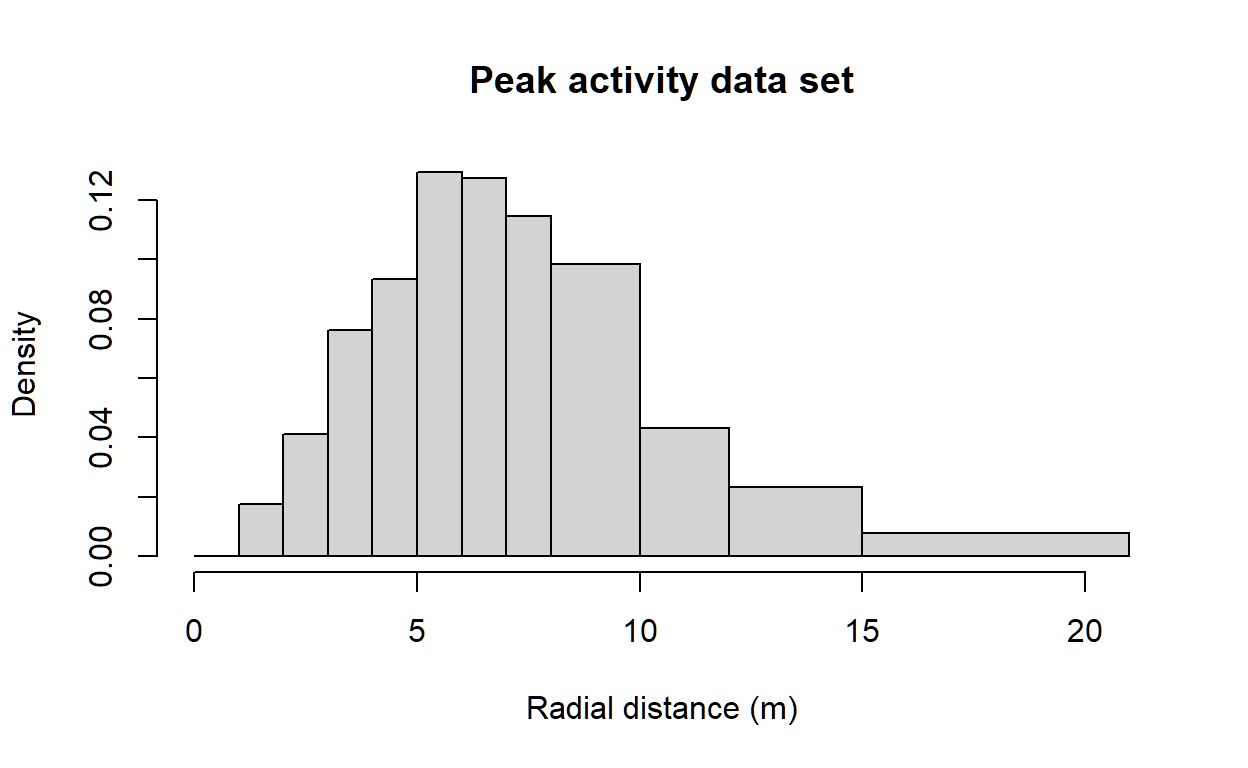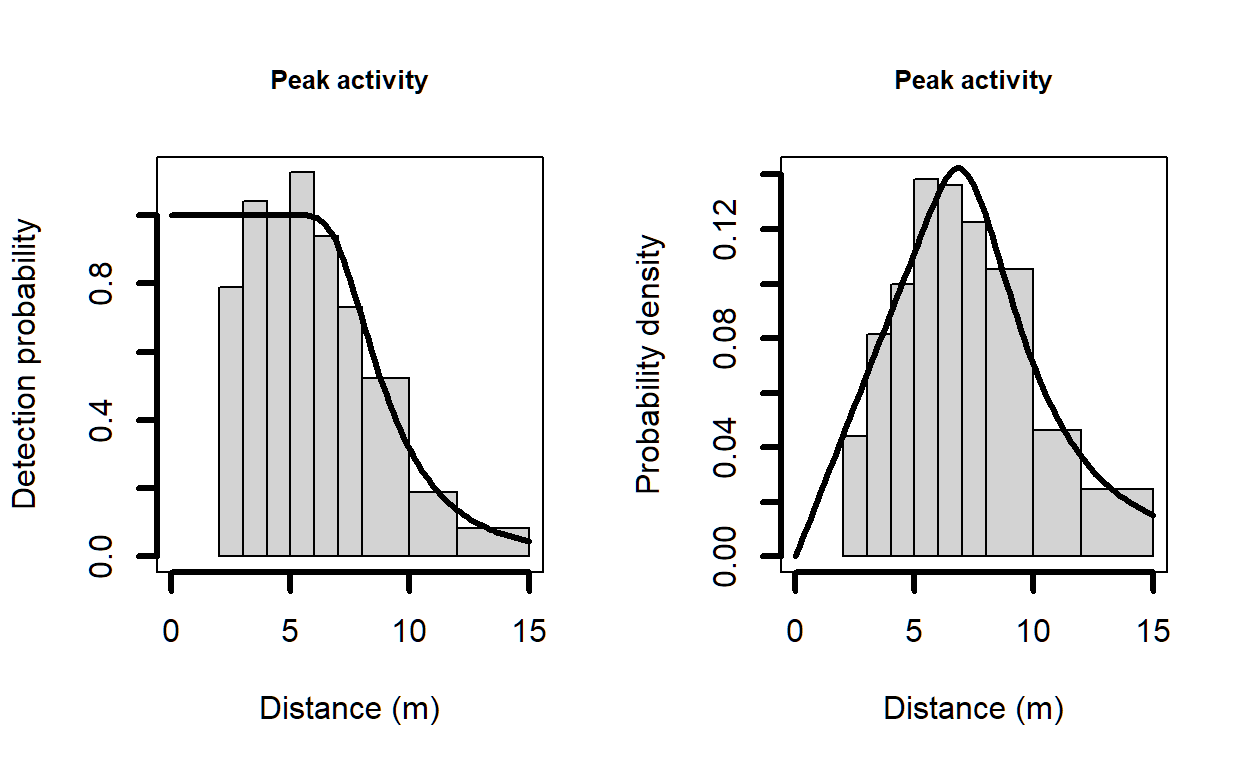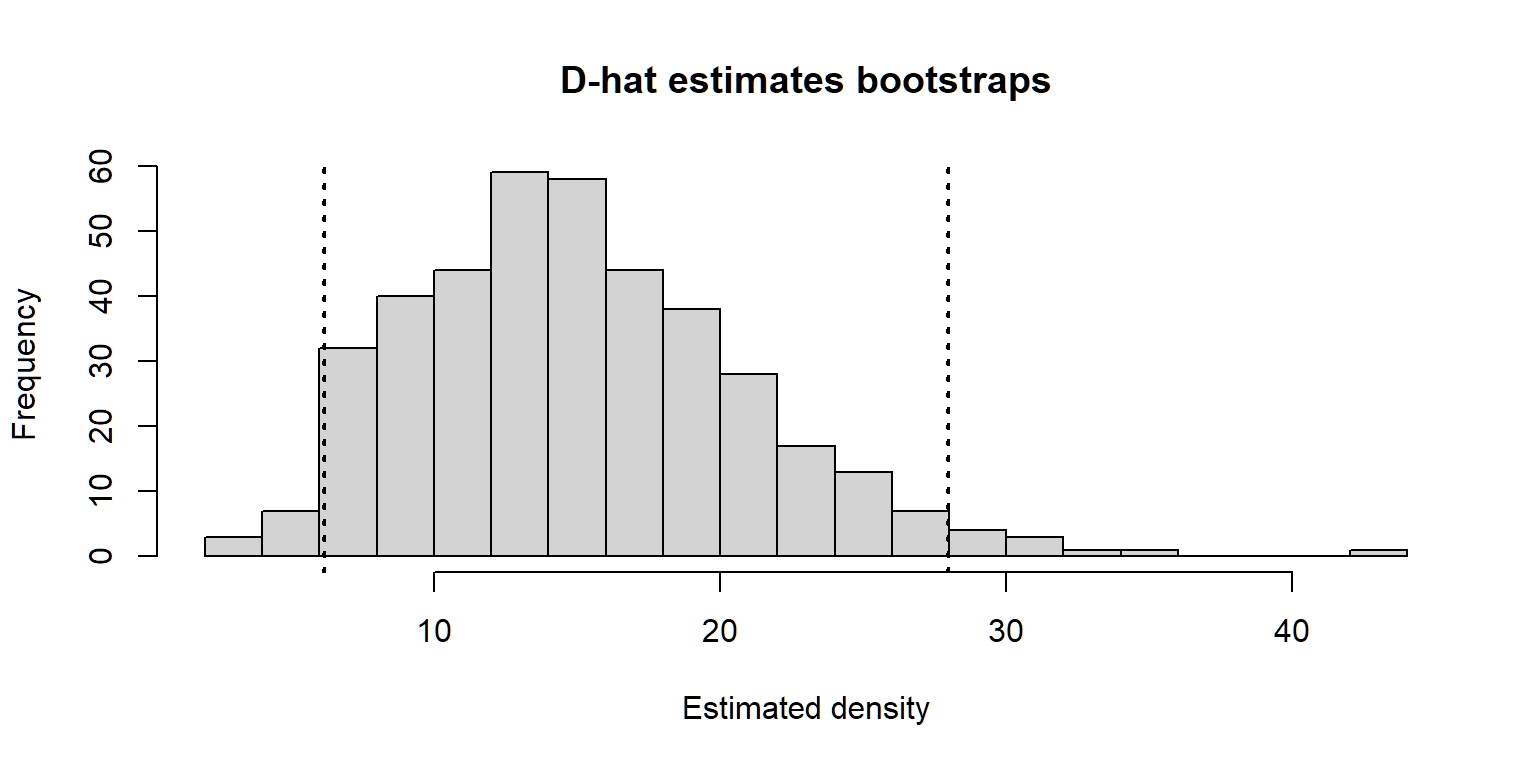# Analysis of camera trapping data

Example analysis with Ivory Coast Maxwell’s duiker.

Eric Howe and Eric Rexstad http://distancesampling.org (CREEM, Univ of St Andrews)https://creem.st-andrews.ac.uk
2020-09-10

# Analysis of camera trapping data using distance sampling

A distance sampling approach to the analysis of camera trapping data offers the potential advantage that individual animal identification is not required. However, accurate animal-to-camera detection distances are required. This requires calibration prior to the survey with images of objects of known size taken at known distances from the camera. See details in Howe, Buckland, Després-Einspenner, & Kühl (2017) for description of the field work and data analysis. Here we present analysis of data from Howe et al. (2017) using the R package Distance Miller, Rexstad, Thomas, Marshall, & Laake (2019).

## Estimating temporal availability for detection

Heat- and motion-sensitive camera traps detect only moving animals within the range of the sensor and the field of view of the camera. Animals are therefore unavailable for detection by camera traps when they are stationary, and when they are above (e.g., semi-arboreal species) or below (e.g., semi-fossorial species) the range of the sensor or the camera, regardless of their distance from the camera in two dimensions. This temporally limited availability for detection must be accounted for to avoid negative bias in estimated densities. When data are abundant, researchers may choose to include only data from times when 100% of the population can be assumed to be active within the vertical range of camera traps Howe et al. (2017). However, for rarely-detected species or surveys with lower effort, it might be necessary to include most or all observations of distance. In these situations, survey duration ($$T_k$$) might be 12- or 24-hours per day, and it becomes necessary to estimate the proportion of time included in $$T_k$$ when animals were available for detection. Methods for estimating this proportion directly from CT data have been described Rowcliffe, Kays, Kranstauber, Carbone, & Jansen (2014), and it can be included in analyses to estimate density Bessone et al. (2020), for example as another multiplier, potentially with an associated standard errors.

## Data input

A data set for recording of detections during peak activity are included in the Distance package. Examine the DuikerCameraTraps described in the data repository Howe, Buckland, Després-Einspenner, Kühl, & Buckland (2018).


library(Distance)
data("DuikerCameraTraps")

## Exploratory Data Analysis

How many transects, how many detections, detection distances


 6274

A1   A2   A3   A4   B1   B2   B3   B4   C1   C2   C3   C4   C5   C6
231   41  444  230    1 1394    8   53   30   97  486   43    1 1398
D3   D4   D5   E3   E4   E5   E6
70  117  258  102    1  375  897 

Note, three sampling stations (B1, C5, E4) had no detections. The one record for each of those stations has distance recorded as NA, but the record is important because it contains effort information.

## Distance recording


breakpoints <- c(seq(0,8,1), 10, 12, 15, 21)
hist(DuikerCameraTraps$distance, breaks=breakpoints, main="Peak activity data set", xlab="Radial distance (m)")## Truncation decisions As described by Howe et al. (2017): a paucity of observations between 1 and 2 m but not between 2 and 3 m, so we left-truncated at 2 m. Fitted detection functions and probability density functions were heavy-tailed when distances >15 m were included, so we right truncated at 15 m. # Detection function fits The conversion factor must be include either in the call to ds() or the call to bootdht(). In this vignette, it is included as an argument to bootdht()  conversion <- convert_units("meter", NULL, "square kilometer") trunc.list <- list(left=2, right=15) peak.hn <- ds(DuikerCameraTraps, transect = "point", key="hn", adjustment=NULL, cutpoints = c(seq(2,8,1), 10, 12, 15), truncation = trunc.list) peak.unicos <- ds(DuikerCameraTraps, transect = "point", key="unif", adjustment = "cos", cutpoints = c(seq(2,8,1), 10, 12, 15), truncation = trunc.list) peak.hr <- ds(DuikerCameraTraps, transect = "point", key="hr", adjustment = NULL, cutpoints = c(seq(2,8,1), 10, 12, 15), truncation = trunc.list) # Model selection  knitr::kable(summarize_ds_models(peak.hn, peak.unicos, peak.hr), digits = 3, caption="Model selection for three key functions fitted to duiker peak activity data") Table 1: Model selection for three key functions fitted to duiker peak activity data Model Key function Formula $$\chi^2$$ $$p$$-value $$\hat{P_a}$$ se($$\hat{P_a}$$) $$\Delta$$AIC 3 Hazard-rate ~1 0 0.398 0.006 0.000 2 Uniform with cosine adjustment terms of order 1,2 NA 0 0.317 0.010 118.185 1 Half-normal ~1 0 0.262 0.005 204.005 Note lack of fit for all models due to over-dispersion. The subject of over-dispersion is described in Howe, Buckland, Després-Einspenner, & Kühl (2019). This overdispersion causes AIC to select overly-complex models, so analysts should specify the number/order of adjustment terms manually when fitting distance sampling models to data from camera traps, rather than allowing automated selection using AIC. As a check of the detection function vis-a-vis Howe et al. (2017), the paper reports the effective detection radius ($$\rho$$) to be 9.4m for the peak activity data set. The effective detection radius can be derived from $$\hat{P_a}$$ as reported by the function ds as $\hat{\rho} = \sqrt{\hat{P_a} \cdot w^2}$  p_a <- peak.hr$ddf$fitted w <- 15 rho <- sqrt(p_a * w^2) $$\hat{P_a}$$ is estimated to be 0.398, resulting in an estimate of $$\hat{\rho}$$ of 9.462. # Selected detection function  par(mfrow=c(1,2)) plot(peak.hr, main="Peak activity", xlab="Distance (m)", showpoints=FALSE, lwd=3, xlim=c(0, 15)) plot(peak.hr, main="Peak activity", xlab="Distance (m)", pdf=TRUE, showpoints=FALSE, lwd=3, xlim=c(0, 15))## Density estimates The camera traps do not view the entire area around them, as would be the case with simple point transect sampling. The portion of the area sampled needs to be incorporated in the estimation of abundance. The data file contains a column multiplier that represents the proportion of the circle sampled. Howe et al. (2017) notes the camera angle of view (AOV) of 42$$^{\circ}$$. The proportion of the circle viewed is this value over 360$$^{\circ}$$. An argument to dht2 is sample_fraction, an obvious place to include this quantity.  viewangle <- 42 # degrees samfrac <- viewangle / 360 conversion <- convert_units("meter", NULL, "square kilometer") peak.hr.dens <- dht2(peak.hr, flatfile=DuikerCameraTraps, strat_formula = ~1, sample_fraction = samfrac, er_est = "P2", convert_units = conversion) print(peak.hr.dens, report="density")  Summary statistics: .Label Area CoveredArea Effort n k ER se.ER cv.ER Total 40.37 1015.748 12317058 5865 21 0 0 0.302 Density estimates: .Label Estimate se cv LCI UCI df Total 14.5106 4.384 0.302 7.8351 26.8734 20.094 Component percentages of variance: .Label Detection ER Total 0.23 99.77 # Bootstrap for variance estimation To produce a more reliable estimate of the precision of the point estimate, produce bootstrap estimates using bootdht. Two issues to note when using bootdht with these camera trap data: • because of the viewing angle of the camera, we must specify the sample_fraction argument just as was done in the call to dht2 • in Howe et al. (2017), estimates of density rather than estimates of abundance are presented. To produce estimates from bootdht consistent with Howe et al. (2017), we write our own simple summary function to extract density estimates from each bootstrap replicate. This is instead of relying upon the default function bootdht_Nhat_summarize provided in the Distance package, which produces estimates of abundance rather than density.  viewangle <- 42 # degrees samfrac <- viewangle / 360 mysummary <- function(ests, fit){ return(data.frame(Dhat = ests$individuals$D$Estimate))
}
duiker.boot.hr <- bootdht(model=peak.hr, flatfile=DuikerCameraTraps, resample_transects = TRUE,
nboot=400, summary_fun=mysummary, sample_fraction = samfrac,
convert.units = conversion)

Confidence limits computed via the percentile method of the bootstrap.


print(summary(duiker.boot.hr))

Bootstrap results

Boostraps          : 400
Successes          : 400
Failures           : 0

median  mean   se  lcl   ucl   cv
Dhat  14.24 15.08 5.81 6.16 27.96 0.41

hist(duiker.boot.hr$Dhat, breaks = 20, xlab="Estimated density", main="D-hat estimates bootstraps") abline(v=quantile(duiker.boot.hr$Dhat, probs = c(0.025,0.975), na.rm=TRUE), lwd=2, lty=3)Figure 1: Distribution of density estimates from bootstrap replicates.

Note the confidence limits computed from the bootstrap are somewhat wider than the confidence limits computed via dht2.

Bessone, M., Kühl, H. S., Hohmann, G., Herbinger, I., N’Goran, K. P., Asanzi, P., … Fruth, B. (2020). Drawn out of the shadows: Surveying secretive forest species with camera trap distance sampling. Journal of Applied Ecology, 57(5), 963–974. https://doi.org/10.1111/1365-2664.13602

Howe, E. J., Buckland, S. T., Després-Einspenner, M.-L., & Kühl, H. S. (2017). Distance sampling with camera traps. Methods in Ecology and Evolution, 8(11), 1558–1565. https://doi.org/10.1111/2041-210X.12790

Howe, E. J., Buckland, S. T., Després-Einspenner, M.-L., & Kühl, H. S. (2019). Model selection with overdispersed distance sampling data. Methods in Ecology and Evolution, 10(1), 38–47. https://doi.org/10.1111/2041-210X.13082

Howe, E. J., Buckland, S. T., Després-Einspenner, M.-L., Kühl, H. S., & Buckland, S. T. (2018). Data from: Distance sampling with camera traps. https://doi.org/https://doi.org/10.5061/dryad.b4c70

Miller, D., Rexstad, E., Thomas, L., Marshall, L., & Laake, J. (2019). Distance sampling in r. Journal of Statistical Software, Articles, 89(1), 1–28. https://doi.org/10.18637/jss.v089.i01

Rowcliffe, J. M., Kays, R., Kranstauber, B., Carbone, C., & Jansen, P. A. (2014). Quantifying levels of animal activity using camera trap data. Methods in Ecology and Evolution, 5(11), 1170–1179. https://doi.org/10.1111/2041-210X.12278